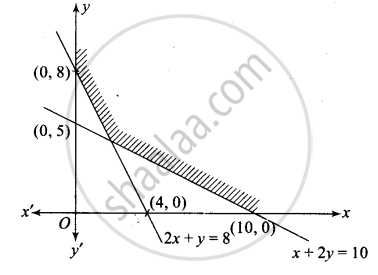# Show that the solution set of the following system of linear inequalities is an unbounded region 2x + y ≥ 8, x + 2y ≥ 10, x ≥ 0, y ≥ 0. - Mathematics

Graph
Sum

Show that the solution set of the following system of linear inequalities is an unbounded region 2x + y ≥ 8, x + 2y ≥ 10, x ≥ 0, y ≥ 0.

#### Solution

We have 2x + y ≥ 8, x + 2 y > 10, x ≥ 0, y ≥ 0

Line 2x + y = 8 passes through the points (0, 8) and (4, 0). Line x + 2y = 10 passes through points (10, 0) and (0, 5).

For (0, 0), 2(0) + (0) – 8 < 0, therefore, the region satisfying the inequality 2x+y ≥ 8 And (0, 0) lie on the opposite side of the line 2x +y = 8.

For (0, 0), (0) + 2(0)- 10 <0.

Therefore, the region satisfying the inequality x + 2y≥ 10 And (0, 0) lie on the opposite side of the line x + 2y = 10. Also, for x ≥ 0, y ≥ 0, region lies in the first quadrant.

The common region is plotted as shown in the following figure.It is clear from the graph that common shaded portion is unbounded.

Concept: Solution of System of Linear Inequalities in Two Variables
Is there an error in this question or solution?

#### APPEARS IN

NCERT Mathematics Exemplar Class 11
Chapter 6 Linear Inequalities
Exercise | Q 18 | Page 109

Share